# Cards

Suppose that are three cards in the hats. One is red on both sides, one of which is black on both sides, and a third one side red and the second black. We are pulled out of a hat randomly one card and we see that one side of it is red. What is the probability that the second side is red?

p =  0.5

### Step-by-step explanation:

$p=1\mathrm{/}2=0.5$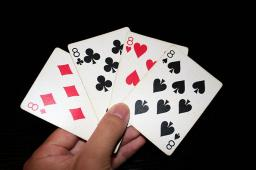Did you find an error or inaccuracy? Feel free to write us. Thank you!Tips to related online calculators
Would you like to compute count of combinations?

## Related math problems and questions:

• White and black ballsThere are 7 white and 3 black balls in an opaque pocket. The balls are the same size. a) Randomly pull out one ball. What is the probability that it will be white? We pull out one ball, see what color it is and return it to the pocket. Then we pull out th
• Two acesFrom a 32 card box we randomly pick 1 card and then 2 more cards. What is the probability that last two drawn cards are aces?
• CardsFrom a set of 32 cards, we randomly pull out three cards. What is the probability that it will be seven kings and ace?
• AceFrom complete sets of playing cards (32 cards), we pulled out one card. What is the probability of pulling the ace?
• Playing cards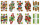From 32 playing cards containing 8 red cards, we choose 4 cards. What is the probability that just 2 will be red?
• BallsFrom the urn in which are 7 white balls and 17 red, gradually drag 3-times without replacement. What is the probability that pulls balls are in order: red red red?
• Monty Hall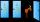Suppose you're on a game show, and you're given the choice of three doors: Behind one door is a car; behind the others, goats. You pick a door, say No. 1, and the host, who knows what's behind the doors, opens another door, say No. 3, which has a goat. Wh
• STRESSED wordEach letter in STRESSED is printed on identical cards, one letter per card, and assembled in random order. Calculate the probability that the cards spell DESSERTS when assembled.
• Red ballsIn the bag there are 3 red, 12 blue and 8 green balls. How many red balls we must be attached to the bag if we want the probability of pulling out the red balls was 20%?
• Hazard game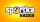In the Sportka hazard game, 6 numbers out of 49 are drawn. What is the probability that we will win: a) second prize (we guess 5 numbers correctly) b) the third prize (we guess 4 numbers correctly)?
• One greenIn the container are 45 white and 15 balls. We randomly select 5 balls. What is the probability that it will be a maximum one green?
• Peaches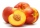There are 20 peaches in the pocket. 3 peaches are rotten. What is the probability that one of the randomly picked two peaches will be just one rotten?
• Green - Red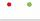We have 5 bags. Each consist one green and 2 red balls. From each we pull just one ball. What is the probability that we doesn't pull any green ball?
• CardsThe player gets 8 cards of 32. What is the probability that it gets a) all 4 aces b) at least 1 ace
• BallsThe urn is 8 white and 6 black balls. We pull 4 randomly balls. What is the probability that among them will be two white?
• Twenty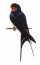Twenty swallows sit on a 10 m long telephone cable. Assume that swallows are completely randomly distributed along the line. (a) What is the probability that more than three swallows sit on a randomly selected section of cable 1 m long? (b) What is the pr
• PIN codePIN on Michael credit card is a four-digit number. Michael told this to his friend: • It is a prime number - that is, a number greater than 1, which is only divisible by number one and by itself. • The first digit is larger than the second. • The second d